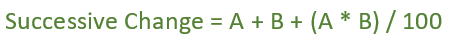Related Articles

# Overall percentage change from successive changes

• Last Updated : 18 Dec, 2018

Given an array Arr which represent the percentage change. The task is to determine the Percentage increase after these percentage change.

Examples:

```Input:  arr[] = {10, 20, 30, 10}
Output: Percentage change is = 88.76 %

Input:  arr[] = {20, 15, 9, 7}
Output: Percentage change is = 60.94 %
```

Solution without successive change:-

let us take a number N = 120.
and percentage changes are given as, arr[] = {10, 20, 30, 10}
Now, if we first increase 120 by 10% i.e 120 * 1.1 We get N = 132
again if we increase it by 20% i.e 132 * 1.2 We get N = 158.4
again if we increase it by 30% i.e 158.4 * 1.3 We get N = 205.92
and lastly if we further increase it by 10% i.e 205.92 * 1.1 We get N = 226.51

Now, Percentage change = (226.51 – 120) / 120 = 0.8876
percentage change = 0.8876 * 100 = 88.76 %

## Recommended: Please try your approach on {IDE} first, before moving on to the solution.How does this formula work?
Let x be the initial value. After A% change, value of x becomes (x + x*A/100)
After successive B% change, value of x becomes (x + x*A/100) + (x + x*A/100)*B/100. So increment in x’s value is x*(A + B + A*B/100)/100. In terms of percentage, we can say that the value is incremented by (A + B + A*B/100)%

Approach:-

1. Simply, Apply sucessive change formula between arr and arr and store the result in result variable
2. Now, calculate successive change between result and arr using above formula and store the result in result variable and so on..

Below is the implementation of the above approach:

## C++

 `// C++ implementation of above approach``#include ``using` `namespace` `std;`` ` `float` `successiveChange(``int` `arr[], ``int` `N)``{``    ``float` `var1, var2, result = 0;`` ` `    ``var1 = arr;``    ``var2 = arr;`` ` `    ``// Calculate successive change of 1st 2 change``    ``result = var1 + var2 + (``float``(var1 * var2) / 100);`` ` `    ``// Calculate successive change``    ``// for rest of the value``    ``for` `(``int` `i = 2; i < N; i++)``        ``result = result + arr[i] + (``float``(result * arr[i]) / 100);`` ` `    ``return` `result;``}`` ` `// Driver code``int` `main()``{``    ``int` `arr[] = {10, 20, 30, 10};``    ``int` `N = ``sizeof``(arr) / ``sizeof``(arr);`` ` `    ``// Calling function``    ``float` `result = successiveChange(arr, N);`` ` `    ``cout << ``"Percentage change is = "` `<< result << ``" %"``;``    ``return` `0;``}`

## Java

 `  ``// Java implementation of above approach`` ` `import` `java.io.*;`` ` `class` `GFG {`` ` ` ` `static` `float` `successiveChange(``int` `arr[], ``int` `N)``{``    ``float` `var1, var2, result = ``0``;`` ` `    ``var1 = arr[``0``];``    ``var2 = arr[``1``];`` ` `    ``// Calculate successive change of 1st 2 change``    ``result = var1 + var2 + ((var1 * var2) / ``100``);`` ` `    ``// Calculate successive change``    ``// for rest of the value``    ``for` `(``int` `i = ``2``; i < N; i++)``        ``result = result + arr[i] + ((result * arr[i]) / ``100``);`` ` `    ``return` `result;``}`` ` `// Driver code``    ``public` `static` `void` `main (String[] args) {``        ``int` `[]arr = {``10``, ``20``, ``30``, ``10``};``    ``int` `N = arr.length;`` ` `    ``// Calling function``    ``float` `result = successiveChange(arr, N);`` ` `    ``System.out.println(``"Percentage change is = "` `+ result + ``" %"``);``    ``}``}``// This code is contributed by shs..`

## Python 3

 `# Python implementation of above approach``def` `successiveChange(arr, N):`` ` `    ``result ``=` `0``;`` ` `    ``var1 ``=` `arr[``0``];``    ``var2 ``=` `arr[``1``];`` ` `    ``# Calculate successive change of 1st 2 change``    ``result ``=` `float``(var1 ``+` `var2 ``+` `            ``(``float``(var1 ``*` `var2) ``/` `100``));``     ` ` ` `    ``# Calculate successive change``    ``# for rest of the value``    ``for` `i ``in` `range``(``2``, N):``        ``result ``=` `(result ``+` `arr[i] ``+``                 ``(``float``(result ``*` `arr[i]) ``/` `100``));`` ` `    ``return` `result;`` ` `# Driver code``arr ``=` `[``10``, ``20``, ``30``, ``10``];``N ``=` `len``(arr) ;`` ` `# Calling function``result ``=` `successiveChange(arr, N);``print` `(``"Percentage change is = %.2f"` `%` `                       ``(result), ``"%"``);`` ` `# This code is contributed ``# by Shivi_Aggarwal``    `

## C#

 `// C# implementation of above approach``using` `System;`` ` `class` `GFG {`` ` ` ` `static` `float` `successiveChange(``int` `[]arr, ``int` `N)``{``    ``float` `var1, var2, result = 0;`` ` `    ``var1 = arr;``    ``var2 = arr;`` ` `    ``// Calculate successive change of 1st 2 change``    ``result = var1 + var2 + ((var1 * var2) / 100);`` ` `    ``// Calculate successive change``    ``// for rest of the value``    ``for` `(``int` `i = 2; i < N; i++)``        ``result = result + arr[i] + ((result * arr[i]) / 100);`` ` `    ``return` `result;``}`` ` `// Driver code``    ``public` `static` `void` `Main () {``        ``int` `[]arr = {10, 20, 30, 10};``    ``int` `N = arr.Length;`` ` `    ``// Calling function``    ``float` `result = successiveChange(arr, N);`` ` `    ``Console.WriteLine(``"Percentage change is = "` `+ result + ``" %"``);``    ``}``}``// This code is contributed by shs..`

## PHP

 ``
Output:
```Percentage change is = 88.76 %
```

Attention reader! Don’t stop learning now. Get hold of all the important mathematical concepts for competitive programming with the Essential Maths for CP Course at a student-friendly price. To complete your preparation from learning a language to DS Algo and many more,  please refer Complete Interview Preparation Course.

My Personal Notes arrow_drop_up